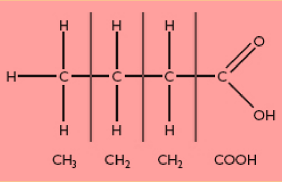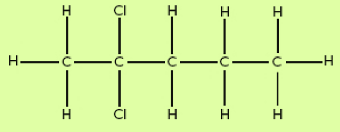# Condensed Structural Formula

In the condensed structural formula, every carbon atom is represented individually, and the following carbon atoms are placed to the atoms that are bonded to that particular carbon atom. Just like in a chemical reaction wherein the first carbon atom has three hydrogen atoms bonded, the second carbon atom has two hydrogen atoms; third carbon atom has three hydrogen atoms, and fourth carbon atom has three hydrogen atoms. Another more condensed way of writing structural formula is when there are two CH2 groups in the middle of the molecule by having (CH2)2 in the middle of the structural formula.

Example 1

Determine the condensed formula of butanoic acid whose structure is as shown below.Solution

Examine the arrangement of atoms and groups of each carbon atom and the combined condensed structural formula is shown below.

CH3CH2CH2COOH

Example 2Below is a structural formula of 2 – dichloropentane. Determine its condensed structural formula.Solution: Single atoms don’t need brackets if more than one is bonded to a carbon atom. Therefore, the condensed structural formula is

CH3CCl2CH2CH2CH3